# Week 4 Lecture 1: Determinants, Eigenvalues, and Eigenvectors

## Determinants

We did examples of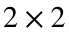determinants in the theory lecture. Lets take a look at an example of a larger determinant.
Consider the matrixwe can take the determinant of this by doing.
A = [1, 4, -1; 3, 2, 0; -1, 4, 3];
det(A)
ans = -44
We can do this with another matrix.
B = [2, 4, 6; 0, 3, 1; 0, 0, -5];
det(B)
ans = -30
Notice that this is just the product of the diagonal. In any triangular matrix the determinant will be the product of its diagonal.

## Eigenvalues and Eigenvectors

Here are some more examples of eigenvalues and eigenvectors. But in the video we will skip to the computation.
The number λ in this equation is called an eigenvalue of M and the vector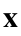is called an eigenvector of M corresponding to λ.
Let's look at a few simple examples. Letand.
We have.
This means that 3 is an eigenvalue of M with corresponding eigenvector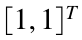. (Remember that the T stands for transpose and just means "make this a column vector.")
Similarly, if we keep the same matrix M but let,
then we have,
so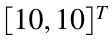is also an eigenvector corresponding to the eigenvalue 3. In fact, it is easy to check that any multiple ofis also an eigenvector with corresponding eigenvalue 3.,
then we have.
This cannot possibly be written as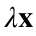because any multiple ofwould have a zero in the first entry. That means that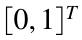is not an eigenvector of M.
It turns out (and you should experiment until you are convinced that this is true) that most vectors are not eigenvectors of M. The only possible eigenvectors are multiples ofand multiples of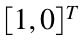. Moreover, there are only two eigenvalues of M. We already saw that 3 is an eigenvalue of M with corresponding eigenvectors that are multiples of. It is also easy to check that 1 is an eigenvalue of M with corresponding eigenvectors that are multiples of. Notice that M was amatrix and we found 2 eigenvalues, each with a family of corresponding eigenvectors. (By "family", I mean that all the eigenvectors were multiples of each other. The word I should really use is "subspace", but we won't go into the technical details of subspaces in this class.) This pattern is not a coincidence. It turns out (although we will not prove it) that every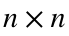matrix has n eigenvalues, and almost everymatrix has n different families of eigenvectors.
There are a couple caveats about this theorem that are worth remembering. First, the rule that there are n eigenvalues of anmatrix works just like the rule that there are n solutions to an nth degree polynomial, which you should already be familiar with from a basic algebra class. In particular, it is possible for eigenvalues to be complex, and it is possible for the same eigenvalue to be repeated multiple times. If one of the eigenvalues is complex, then its corresponding eigenvectors can also be written with complex entries. If an eigenvalue is repeated k times, then it will (almost always) have k different families of eigenvectors. It is actually possible for repeated eigenvalues to share the same family of eigenvectors (which is where the "almost" in our theorem comes from). We won't worry about that issue in our class, because it is quite rare and makes the analysis much more complicated.
The course videos show how to find eigenvalues and eigenvectors of amatrix by hand. It is also possible to use that method for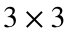matrices (but it is much more difficult). For anything larger than amatrix it is not usually possible to find eigenvalues/eigenvectors by hand. Since we are primarily interested in large matrices, we will not worry about finding these values on paper. Instead, we will let MATLAB do the hard work for us.
In MATLAB, we can find the eigenvalues and eigenvectors of a matrix M with the eig command. This command has many different modes, and you should (as always) investigate the help file with doc eig to learn more about it. We will only use two of these modes. If you ask for only one output, such as
M = [1 2; 0 3];
eig(M)
ans = 2×1
1 3
then MATLAB returns a vector with all n eigenvalues of M.
We can also ask MATLAB for the eigenvalue decomposition or the "S, Lambda, S inverse", which gives us both the eigenvalues (saved in the diagonal of D) and the corresponding eigenvectors (saved in the respective columns of V). Notice that MATLAB always return an eigenvector of length (distance from the origin) of unity.
[V, D] = eig(M)
V = 2×2
1.0000 0.7071 0 0.7071
D = 2×2
1 0 0 3
then each column of the matrix V will be an eigenvector of M and each diagonal entry of D will be the corresponding eigenvalue. (That is, D(i, i) is the eigenvalue corresponding to the eigenvector V(:, i).)
Lets now look at the plot from the theory lecture.
A = [4, 1; 3, 2];
[V, D] = eig(A);
v1 = V(:,1); v2 = V(:,2);
v1_x = [0:v1(1)/100:v1(1)];
v1_y = [0:v1(2)/100:v1(2)];
v2_x = [0:v2(1)/100:v2(1)];
v2_y = [0:v2(2)/100:v2(2)];
subplot(2,2,1);
plot(v1_x, v1_y, v2_x, v2_y, 'Linewidth', 4)
v1 = A*v1; v2 = A*v2;
v1_x = [0:v1(1)/100:v1(1)];
v1_y = [0:v1(2)/100:v1(2)];
v2_x = [0:v2(1)/100:v2(1)];
v2_y = [0:v2(2)/100:v2(2)];
subplot(2,2,2);
plot(v1_x, v1_y, '--', v2_x, v2_y, '--', 'Linewidth', 4)
v = randn(2,1);
v_x = [0:v(1)/100:v(1)];
v_y = [0:v(2)/100:v(2)];
subplot(2,2,3)
plot(v_x, v_y, 'Linewidth',4)
v = A*v;
v_x = [0:v(1)/100:v(1)];
v_y = [0:v(2)/100:v(2)];
subplot(2,2,4)
plot(v_x, v_y, '--', 'Linewidth',4)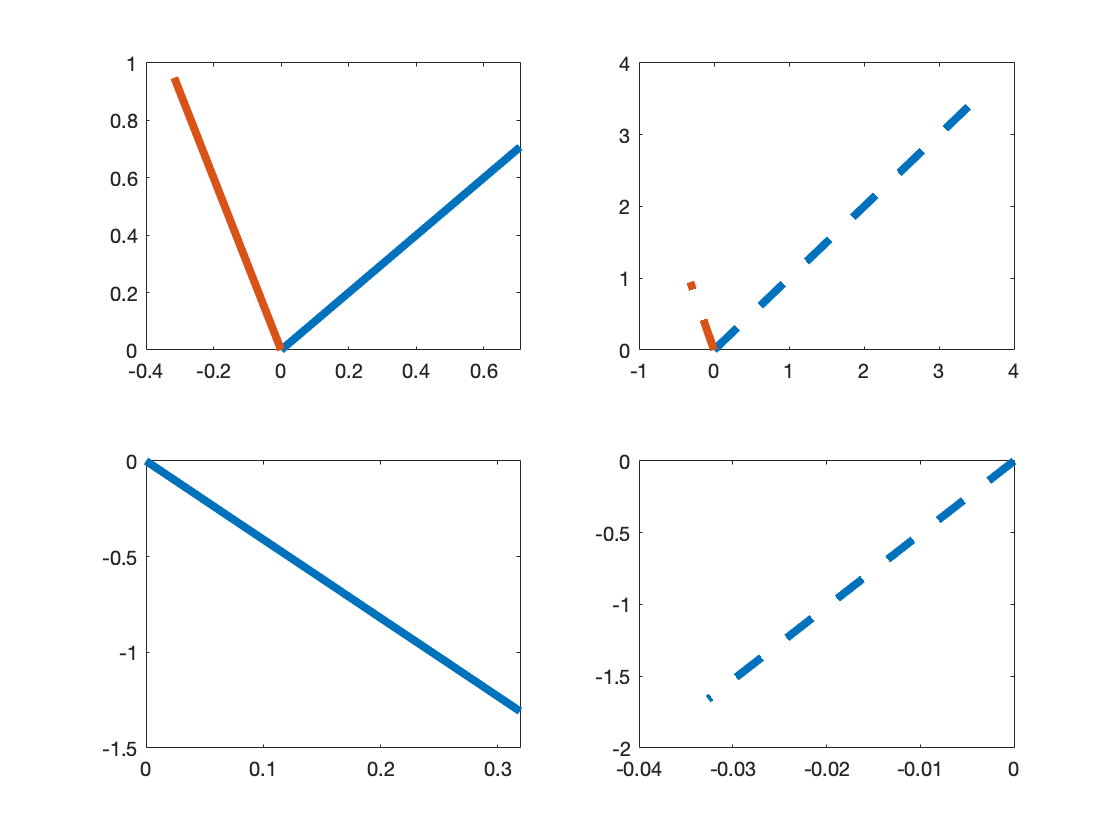#### A word on eigendecomposition

MATLAB does not just use this format because it's a convenient way to store all of the eigenvectors; the matrices V and D have important mathematical significance. It turns out that we can rewrite the matrix M using V and D as follows: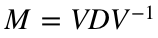.
(We will verify this in MATLAB but not prove it.) This formula is called an eigendecomposition of M. You can check the formula in Matlab with
[V, D] = eig(M)
V = 2×2
1.0000 0.7071 0 0.7071
D = 2×2
1 0 0 3
V*D*inv(V)
ans = 2×2
1 2 0 3
It is worth noting that the eigenvectors and eigenvalues of a matrix might be complex, so there might be lots of imaginary numbers on the right side of the above equation. We won't worry about these complex numbers for two reasons: First, they all cancel out when you multiply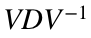, so there won't be any complex numbers in our final answers. Second, we will ultimately only care about the magnitude of eigenvalues, so we will only really work with real numbers. (Remember, the magnitude of a complex number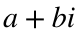is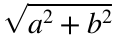. You can use the MATLAB command abs to find the magnitude of a complex number.)
The eigendecomposition is particularly useful for computing powers of M. To see this, notice that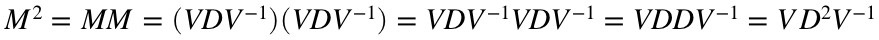,
because the innermost V and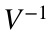cancel out. (It is important to remember that you cannot reorder matrix multiplication, so we are only allowed to cancel aand V if they appear beside each other.)
Similarly,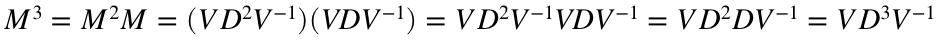.
The pattern should quickly become apparent. In general, we have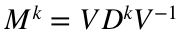.
The reason this is so convenient is that D is a diagonal matrix and it is easy to compute powers of a diagonal matrix: We just raise each entry of the matrix to the same power. For example,.